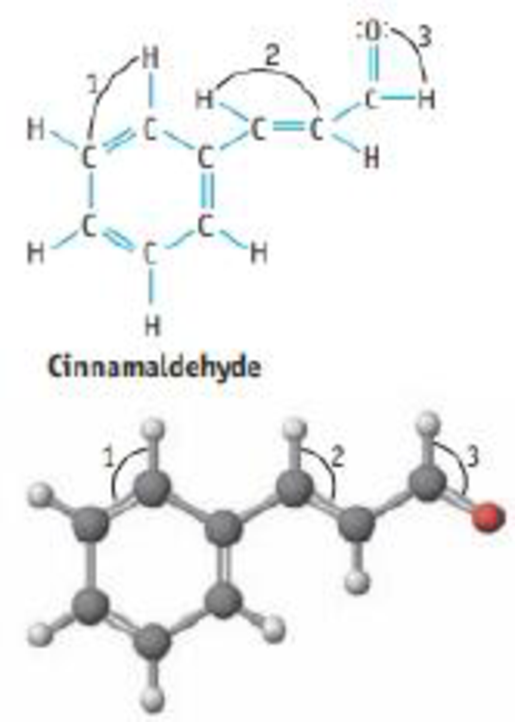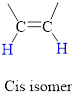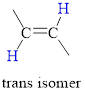Chapter 9, Problem 41GQ

Chapter
Section
Textbook Problem

Cinnamaldehyde ocaus naturally in cinnamon oil.(a) What is the most polar bond in the molecule?(b) How many σ bonds and how many π bonds are there?(c) Is cis-trans isomerism possible? If so, draw the isomers of the molecule.(d) Give the hybridization of the C atoms in the molecule.(e) What are the values of the bond angles 1, 2, and 3 ?

(a)

Interpretation Introduction

Interpretation:

The most polar bond in the given compound has to be identified.

Concept Introduction:

Polar covalent bond involves an unequal sharing of electrons between two bonded atoms; it creates a partial separation of charge and a dipole moment.

Covalent bonds are polar, if there is a difference in electronegativity between the bonded atoms.

Explanation

The structure of the given molecule cinnamaldehyde is,

This molecule contain one (-CHO) g...

(b)

Interpretation Introduction

Interpretation:

The number of σandπ bonds in the given compound has to be determined.

Concept Introduction:

Sigma (σ) bonds are the bonds in which shared hybrid orbital’s electron density are concentrated along the internuclear axis.

Pi (π) bonds are the bonds in which shared unhybridized orbital’s (p, d…) electron density are concentrated in above and below of the plane of the molecule.

TypeofBondNo.ofσbondsNo.ofπbondsSingle10Double11Triple12

(c)

Interpretation Introduction

Interpretation:

If cis and Trans isomerism possible for the given molecule, then the isomers should be drawn.

Concept Introduction:

Isomer: The molecule has same molecular formula and attached for different functional groups it is called isomers.

Cis Isomer: The two groups attached on same side of C=C double bond irrespective of molecules or atoms is called cis isomer this type molecule has polar nature.

In cis isomer the hydrogen atoms are on the same side of the two carbon atom.Trans isomer: The two groups are attached on opposite sides of the carbon-carbon double bond.

The hydrogen atoms are on the opposite side of the two carbon atom.(d)

Interpretation Introduction

Interpretation:

Hybridization of carbon atom in the molecule should be determined.

Concept Introduction:

Hybridization is the mixing of valence atomic orbitals to get equivalent hybridized orbitals that having similar characteristics and energy.

Geometry of different types of molecule with respect to the hybridizations is mentioned are mentioned below,

TypeofmoleculeHybridaizationAtomicorbitalsusedforhybridaizationGeometryAX2sp1s+1pLinearAX3,AX2Bsp21s+2pTrigonalplanarAX4,AX3B,AX2B2sp31s+3pTetrahedralAX5,AX4B,AX3B2,AX2B3sp3d1s+3p+1dTrigonalbipyramidalAX6,AX5B,AX4B2sp3d21s+3p+2dOctahedralACentralatomXAtomsbondedtoABNonbondingelectronpairsonA

(e)

Interpretation Introduction

Interpretation:

The respective bond angles (1,2 and 3) in the given molecule should be determined.

Concept Introduction:

Hybridization is the mixing of valence atomic orbitals to get equivalent hybridized orbitals that having similar characteristics and energy.

Bond angle: The bond angle is the angle formed between three atoms at least two bonds.

TypeofmoleculeHybridaizationGeometryBondangleAX2spLinear180°AX3,AX2Bsp2Trigonalplanar120°AX4,AX3B,AX2B2sp3Tetrahedral109.5°AX5,AX4B,AX3B2,AX2B3sp3dTrigonalbipyramidal120°,90°AX6,AX5B,AX4B2sp3d2Octahedral90°ACentralatomXAtomsbondedtoABNonbondingelectronpairsonA

Still sussing out bartleby?

Check out a sample textbook solution.

See a sample solution

The Solution to Your Study Problems

Bartleby provides explanations to thousands of textbook problems written by our experts, many with advanced degrees!

Get Started

Find more solutions based on key concepts